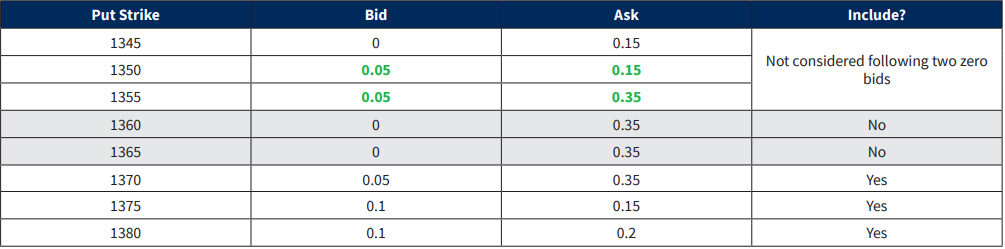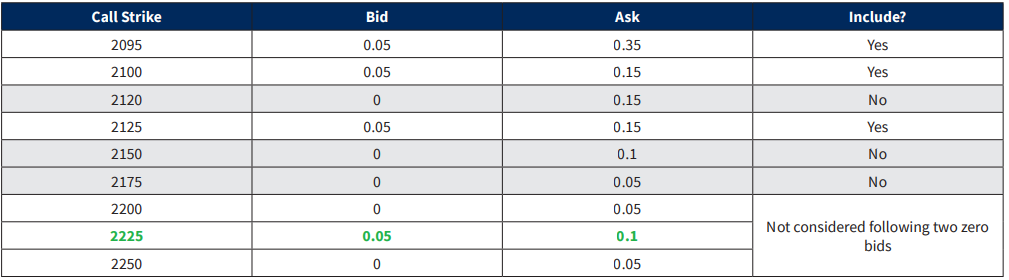## VIX 历史简介

1993年，CBOE Global Markets，InCorporation®(CBOE®) 推出了 CBOE 波动率指数®(VIX®Index)，该指数最初旨在衡量市场对现货标准普尔100®指数（OEX®Index）期权价格所隐含的 30 天波动率的预期。波动率指数很快成为美国股市波动的首要基准。它经常出现在华尔街日报、Barron‘s 和其他领先的金融出版物上，以及 CNBC、彭博电视 (Bloomberg TV) 和 CNN/Money 的商业新闻节目中，在这些节目中，VIX 指数通常被称为“恐慌指数”。

10 年后的 2003 年，芝加哥期权交易所（CBOE）与高盛（Goldman Sachs）一起更新了波动率指数(VIX Index)，以反映一种衡量预期波动率的新方法，这种方法继续被金融理论家、风险经理和波动率交易员广泛使用。

2014 年，CBOE 增强了 VIX 指数，将 SPX WeeklysSM 系列纳入其中。CBOE 于 2005 年首次推出周度期权，目前可在数百种指数、股票、ETF和ETN上使用周度期权，并已成为一种非常受欢迎和交易活跃的风险管理工具。今天，SPX 周度期权占所有 SPX 期权交易的三分之一，平均每天交易近 350000 份合约。

CBOE 于 2016 年 4 月开始在美国交易时间以外发布 VIX 指数。波动率指数现在可以在凌晨 3 点之间的 “extended trading hours”（美东时间 3:00 a.m. 到 9：15 a.m.）内交易，也可以在正常交易时段（美东时间 9：30 a.m. 到 4：15 p.m.）交易。作为波动率指数扩展的一部分，CBOE 对“extended trading hours”和正常交易时段内的波动率指数值实施了平滑算法。

2004 年 3 月 24 日，CBOE 在其全新的全电子 CBOE Futures ExchangeSM(CFE®)上推出了首个交易所交易的波动率指数期货合约。两年后的 2006年2月，CBOE 推出了波动率指数期权，这是 CBOE 历史上最成功的新产品。2015 年，波动率指数期权和期货的总交易活动增长到每天近 80 万份合约。

### VIX & VXO 波动率指数的历史价格

tips：VXO 是 CBOE 编制的另一个波动率指数，选择 S&P100 指数期权的隐含波动率为编制基础。

## VIX 的计算

VIX 的计算公式是：

$$\sigma^{2} = \frac{2}{T} \sum_{i} \frac{\Delta K_{i}}{K_{i}^{2}}\times e^{RT}\times Q(K_{i}) – \frac{1}{T} \times (\frac{F}{K_{0}} – 1)^{2}$$

$$VIX = \sigma \times 100$$

• T: 距离到期日剩余时间
• F：由指数期权价格确定的 SPX 远期价格水平
• $$K_{0}$$：F 这个数下面最近的一档行权价
• Ki：第 i 个虚值期权的价格（如果 $$K_{i} > K_{0}$$，就看 call；如果 $$K_{i} < K_{0}$$，就看 put；如果 $$K_{i} = K_{0}$$，call 和 put 都要看）
• $$\Delta K_{i}$$ ：行权价间距（两侧行权价之差的一半）
• $$\Delta K_{i} = \frac{K_{i+1} – K_{i-1}}{2}$$
• R ：距离到期日的无风险利率
• $$Q(K_{i})$$：$$K_{i}$$ 为行权价的期权的 bid 和 ask 价格的中点价

VIX 指数衡量的是标普 500 指数未来 30 天的预期波动率。VIX 指数的成分股是NEAR 和 NEXT 两个期限的期权链，NEAR 和 NEXT 期权的距离到期日剩余天数都在 23 天到 37 天之间

### 距离到期日剩余时间 T

$$T = (M_{CurrentDay} + M_{SettlementDay} + M_{OtherDays})$$ /Minutes in a year

• $$M_{CurrentDay}$$ ：当天距离 24：00 剩余的分钟数
• $$M_{SettlementDay}$$ ：
• 如果是月度期权，就是美东时间 0：00 a.m. 到 9：30 a.m. 的分钟数
• 如果是周度期权，就是美东时间 0：00 a.m. 到 4：30 p.m. 的分钟数
• $$M_{OtherDays}$$：当天到到期日中间的分钟数（不含当天的半天和到期日的半天）

• $$T_{1} = (854 + 510 + 34560)/ 525600$$
• $$T_{2} = (854 + 900 + 44640)/ 525600$$

### 计算 SPX 远期价格水平 F

F = Strike Price + $$e^{RT} \times$$ (Call Price – Put Price)

$$F_{1} = 1965 + e^{0.000305\times 0.0683486}\times (21.05-23.15) = 1962.89996$$

$$F_{2} = 1960 + e^{0.000286\times 0.0882686}\times (27.30-24.90) = 1962.40006$$

### 计算 F 这个数下面最近的一档行权价$$K_{0}$$筛选 OTM put筛选 OTM call

### 计算行权价间距 $$\Delta K_{i}$$

$$\Delta K_{i}$$就是两侧行权价之差的一半。

$$\Delta K_{i} = \frac{K_{i+1} – K_{i-1}}{2}$$

### 代入计算波动率

$$\sigma_{1}^{2}=\frac{2}{T_{1}} \sum_{i} \frac{\Delta K_{i}}{K_{i}^{2}}\times e^{R_{1}T_{1}}\times Q(K_{i})-\frac{1}{T_{1}} \times (\frac{F_{1}}{K_{0}} – 1)^{2}=0.018495–0.00003203=0.01846292$$

$$\sigma_{2}^{2} = \frac{2}{T_{1}} \sum_{i} \frac{\Delta K_{i}}{K_{i}^{2}}\times e^{R_{2}T_{2}}\times Q(K_{i}) – \frac{1}{T_{2}} \times (\frac{F_{2}}{K_{0}} – 1)^{2} = 0. 018838 – 0. 00001699 = 0.01882101$$

### 计算 VIX

$$VIX = 100\times \sqrt{(T_{1}\sigma_{1}^{2}\times \frac{N_{T_{2}}-N_{30}}{N_{T_{2}}-N_{T_{1}}} – T_{2}\sigma_{2}^{2}\times \frac{N_{30}-N_{T_{1}}}{N_{T_{2}}-N_{T_{1}}} )\times \frac{N_{365}}{N_{30}}}$$

• $$N_{T_{1}}$$ ：NEAR 期权距离到期日剩余分钟数 (35924)
• $$N_{T_{2}}$$ ：NEXT 期权距离到期日剩余分钟数 (46394)
• $$N_{30}$$：30 天的总分钟数 (30 x 1440 = 43200)
• $$N_{365}$$：365 天的总分钟数 (365 x 1440 = 525600)

VIX = 100 × 0.13685821 = 13.69

## 写在最后

tips：博主已经把相关的代码写好，只要换一下标的证券，就可以轻松生成其他证券的波动率指数。如果你有兴趣，欢迎加博主 QQ 哦。